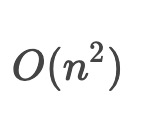# 算法之时间复杂度

`T(n)=Ｏ(f(n))`中存在一个常数`C`，使得在当n趋于正无穷时`T(n)≤C*f(n)`。简单来说，就是T(n)在n趋于正无穷时最大也就跟f(n)差不多大。也就是说当n趋于正无穷时`T(n)`的上界是`C*f(n)`。其虽然对f(n)没有规定，但是一般都是砍掉常数，系数。

``````for(\$i = 1;\$i <= \$n;\$i++){
\$x++;
}

for(\$i = 1;\$i <= \$n;\$i++){
for (\$j = 1;\$j <= \$n;\$j++){
\$x++;
}
}``````----------end

tag(s): none
show comments · back · home